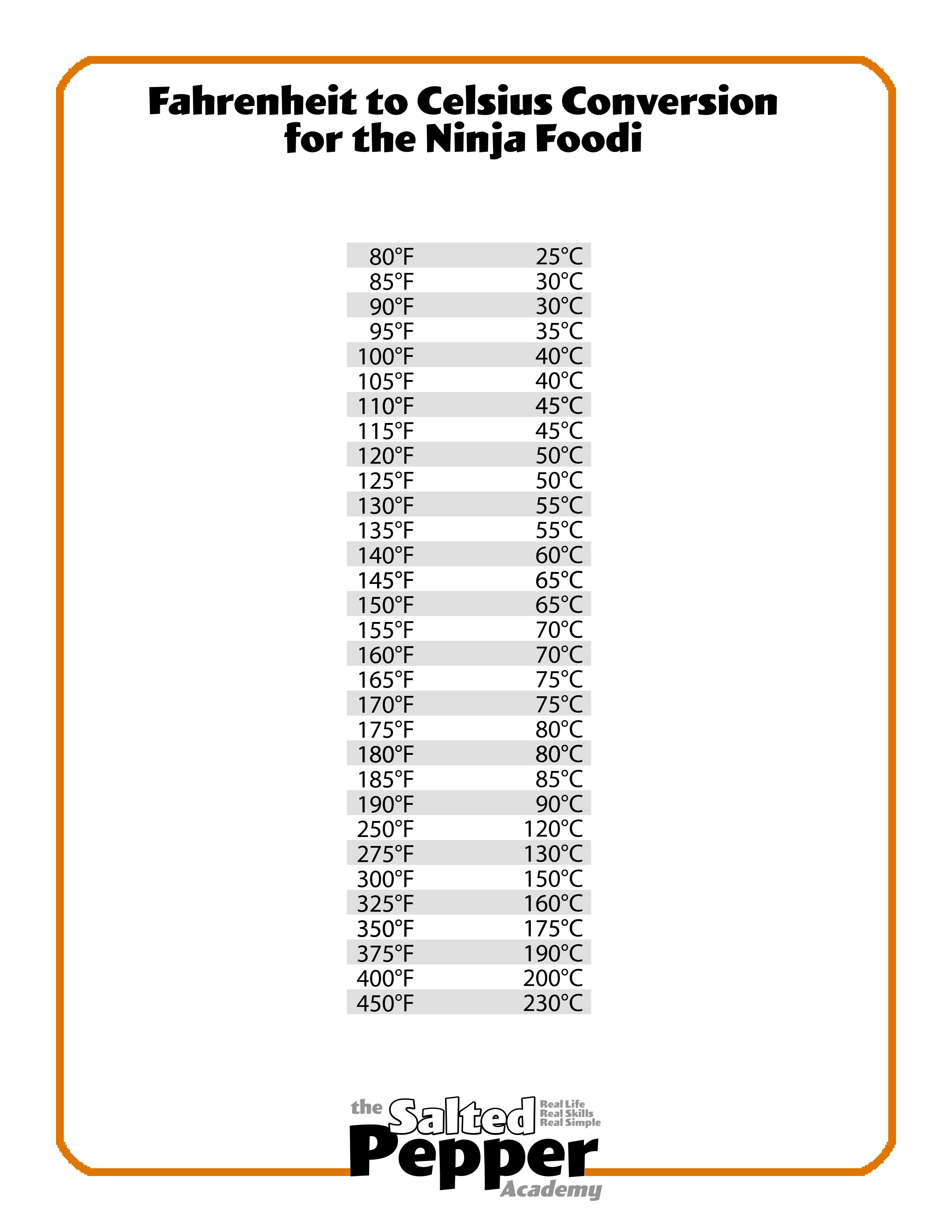# What are the Temperatures Conversions from Fahrenheit to Celsius?

There is a precise formula for converting Fahrenheit to Celsius and vice versa, but it involves some math, including fractions. Here is a slightly easier way, but it still involves a little math.

Celsius to Fahrenheit:

Multiply the Celsius temperature by 1.8 and add 32.

Let's say my celsius temperature is 180℃, my formula would look like this:

180x1.8+32= 356℉

### Fahrenheit to Celsius:

You can do this in reverse to calculate Fahrenheit to Celsius and the formula looks like this. Subtract 32 from the Fahrenheit temperature and divide by 1.8.

Let's say my Fahrenheit temperature is 350℉, my formula would look like this:

350-32=318

318/1.8=176.6℃

You can also use Google, which is what I do all the time when converting temperatures in my recipes.

It's super easy to use, just plug in the temperature and it will auto calculate.

### Conversions & Cooking

When cooking and converting temperatures, they aren't always going to be exact. Most ovens that use Fahrenheit do not have a setting for 356℉, so you would use the closest temperature. In this example it would be 350℉, unless your stove has the ability to be set at 360℉, then I might use that instead.

When a temperature falls right in the middle of two setting you have on your appliance, there are times when I would pick the lower temperature and times when I would pick the higher temperature. There isn't any right or wrong here, so don't worry too much about it. Some instances where I might round down the number instead of rounding up are when baking things where I want the center to get done before the outside browns too much, like a cornbread or a cake. Some instances where I might round up instead of down would be when I want more browning and heat on the outside, like when cooking most meats.

Here is a basic conversion chart with the conversions already done and rounded up or down based on the settings that the Ninja Foodi Pressure Cooker & Air Crisper has. This can be used for any appliance, just use the temperature that's closest to the one listed. When in doubt, I would go down to the lower temp rather than go up to the higher temp. You can also download this chart right here: Temperature Conversion Chart